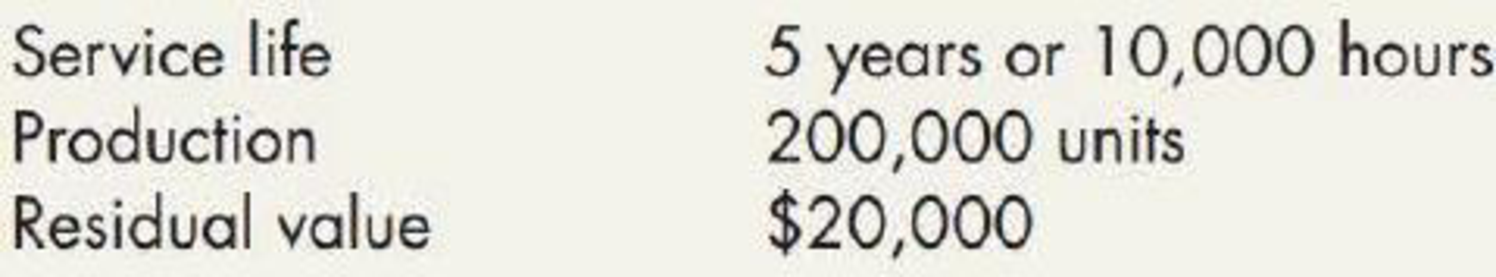Chapter 11, Problem 1E### Intermediate Accounting: Reporting...

3rd Edition
James M. Wahlen + 2 others
ISBN: 9781337788281

#### Solutions

Chapter
Section### Intermediate Accounting: Reporting...

3rd Edition
James M. Wahlen + 2 others
ISBN: 9781337788281
Textbook Problem
172 views

# Depreciation Methods Gruman Company purchased a machine for $220,000 on January 2, 2019. It made the following estimates:In 2019, Gruman uses the machine for 1,800 hours and produces 44,000 units. In 2020, Gruman uses the machine for 1,500 hours and produces 35,000 units.Required: 1. Compute the depreciation expense for 2019 and 2020 under each of the following methods: a. straight-line b. sum-of-the-years’-digits (round to the nearest dollar) c. double-declining-balance d. activity method based on hours worked e. activity method based on units of output 2. For each method, what is the book value of the machine at the end of 2019? At the end of 2020? 3. Next Level If Gruman used a service life of 8 years or 15,000 hours and a residual value of$10,000, what would be the effect on (a) depreciation expense and (b) book value under the straight-line, sum-of-the-years’- digits, and double-declining-balance depreciation methods?

1.

To determine

Compute the depreciation expense of Company G for 2019 and 2020 under the given depreciation method.

Explanation

Depreciation: Depreciation is a method of reducing the capitalized cost of long-lived operating assets or plant assets for the period the asset is used.

Straight-line depreciation method: The depreciation method which assumes that the consumption of economic benefits of long-term asset could be distributed equally throughout the useful life of the asset is referred to as straight-line method.

Activity method:  In this method of depreciation, the amount of depreciation is charged based on the units of production or hours worked each year. When the usefulness of an asset is related to the production cost of a unit, this method is more appropriate to use.

Sum-of- the-years’ digits method:  Sum-of-the years’ digits method determines the depreciation by multiplying the depreciable base and declining fraction.

Double-declining-balance method: The depreciation method which assumes that the consumption of economic benefits of long-term asset is high in the early years but gradually declines towards the end of its useful life is referred to as double-declining-balance method.

a. Straight line method:

2019:

Depreciation expenses=Acquisition cost – Residual valueUseful life =$220,000$20,0005 years=$40,000 2020: Depreciation expenses=Acquisition cost – Residual valueUseful life =$220,000$20,0005 years=$40,000

b. Sum-of- the-years’ digits method:

 Year Depreciable Base  ($) (1) Depreciation rate per year Depreciation expense ($) 2019 200,000 × 515 = 76,471 2020 200,000 × 415 = 71,691

Table (1)

Working note (1):

Calculate the depreciable base of asset.

Depreciable base = Purchase price –Residual value = $220,000–$20,000=$200,000 Working note (2): Calculate the denominator of the fraction for sum-of-the-year’s digit. Sum-of-the-digits = n×(n+1)2=5×(5+1)2=5×62=15 c 2. To determine Calculate the book value of the machine at the end of 2019 and 2020 under each depreciation method. 3. To determine Identify the effect of (a) the depreciation expense and (b) book value under the straight line, sum of the year’s digit, and double-declining balance method, assume that Company G used a service life of 8 years, or 15,000 hours and residual value of$10,000.

### Still sussing out bartleby?

Check out a sample textbook solution.

See a sample solution

#### The Solution to Your Study Problems

Bartleby provides explanations to thousands of textbook problems written by our experts, many with advanced degrees!

Get Started